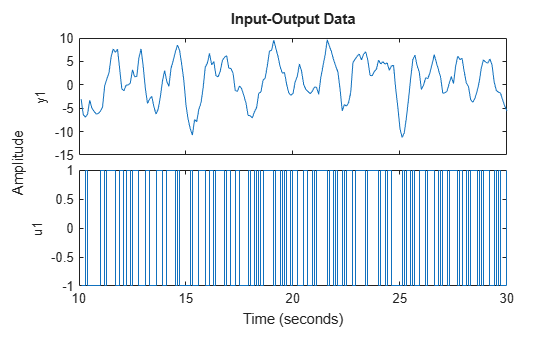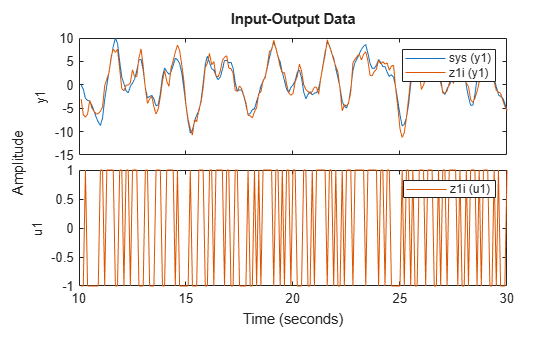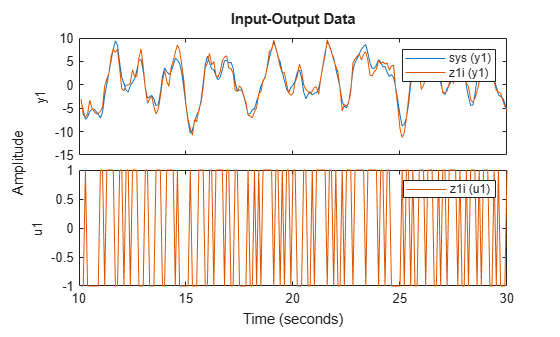# simOptions

Option set for `sim`

## Syntax

``opt = simOptions``
``opt = simOptions(Name,Value)``

## Description

example

````opt = simOptions` creates the default option set for `sim`.```

example

````opt = simOptions(Name,Value)` creates an option set with the options specified by one or more `Name,Value` pair arguments.```

## Examples

collapse all

`opt = simOptions;`

Create an option set for `sim` specifying the following options.

• Zero initial conditions

• Input offset of 5 for the second input of a two-input model

`opt = simOptions('InitialCondition','z','InputOffset',[0; 5]);`

Create noise data for a simulation with `500` input data samples and two outputs.

`noiseData = randn(500,2);`

Create a default option set.

`opt = simOptions;`

Modify the option set to add the noise data.

```opt.AddNoise = true; opt.NoiseData = noiseData;```

Use historical input-output data as a proxy for initial conditions when simulating your model.

Load a two-input, one-output data set.

`load iddata7 z7`

Identify a fifth-order state-space model using the data.

`sys = n4sid(z7, 5);`

Split the data set into two parts.

```zA = z7(1:15); zB = z7(16:end);```

Simulate the model using the input signal in `zB`.

`uSim = zB;`

Simulation requires initial conditions. The signal values in `zA` are the historical data, that is, they are the input and output values for the time immediately preceding data in `zB`. Use `zA` as a proxy for the required initial conditions.

```IO = struct('Input',zA.InputData,'Output',zA.OutputData); opt = simOptions('InitialCondition',IO);```

Simulate the model.

`ysim = sim(sys,uSim,opt);`

To understand how the past data is mapped to the initial states of the model, see Understand Use of Historical Data for Model Simulation.

```load iddata1ic z1i plot(z1i)```Examine the initial value of the output data `y(1)`.

`ystart = z1i.y(1)`
```ystart = -3.0491 ```

The measured output does not start at 0.

Estimate a second-order transfer function `sys` and return the estimated initial condition `ic`.

```[sys,ic] = tfest(z1i,2,1); ic```
```ic = initialCondition with properties: A: [2x2 double] X0: [2x1 double] C: [0.2957 5.2441] Ts: 0 ```

`ic` is an `initialCondition` object that encapsulates the free response of `sys`, in state-space form, to the initial state vector in `X0`.

Simulate `sys` using the estimation data but without incorporating the initial conditions. Plot the simulated output with the measured output.

```y_no_ic = sim(sys,z1i); plot(y_no_ic,z1i) legend('Model Response','Output Data')```The measured and simulated outputs do not agree at the beginning of the simulation.

Incorporate the initial condition into the `simOptions` option set.

```opt = simOptions('InitialCondition',ic); y_ic = sim(sys,z1i,opt); plot(y_ic,z1i) legend('Model Response','Output Data')```The simulation combines the model response to the input signal with the free response to the initial condition. The measured and simulated outputs now have better agreement at the beginning of the simulation. This initial condition is valid only for the estimation data `z1i`.

## Input Arguments

collapse all

### Name-Value Pair Arguments

Specify optional comma-separated pairs of `Name,Value` arguments. `Name` is the argument name and `Value` is the corresponding value. `Name` must appear inside quotes. You can specify several name and value pair arguments in any order as `Name1,Value1,...,NameN,ValueN`.

Example: `'AddNoise',true','InputOffset',[5;0]` adds default Gaussian white noise to the response model and specifies an input offset of `5` for the first of two model inputs.

Simulation initial conditions, specified as one of the following:

• `'z'` — Zero initial conditions.

• Numerical column vector of initial states with length equal to the model order.

For multiexperiment data, specify a matrix with Ne columns, where Ne is the number of experiments, to configure the initial conditions separately for each experiment. Otherwise, use a column vector to specify the same initial conditions for all experiments.

Use this option for state-space models (`idss` and `idgrey`) only.

• `initialCondition` object — `initialCondition` object that represents a model of the free response of the system to initial conditions. For multiexperiment data, specify a 1-by-Ne array of objects, where Ne is the number of experiments.

Use this option for linear models only. For an example, see Obtain and Apply Estimated Initial Conditions.

• Structure with the following fields, which contain the historical input and output values for a time interval immediately before the start time of the data used in the simulation:

FieldDescription
`Input`Input history, specified as a matrix with Nu columns, where Nu is the number of input channels. For time-series models, use `[]`. The number of rows must be greater than or equal to the model order.
`Output`Output history, specified as a matrix with Ny columns, where Ny is the number of output channels. The number of rows must be greater than or equal to the model order.

For an example, see Use Historical Data to Specify Initial Conditions for Model Simulation.

For multiexperiment data, configure the initial conditions separately for each experiment by specifying `InitialCondition` as a structure array with Ne elements. To specify the same initial conditions for all experiments, use a single structure.

The software uses `data2state` to map the historical data to states. If your model is not `idss`, `idgrey`, `idnlgrey`, or `idnlarx`, the software first converts the model to its state-space representation and then maps the data to states. If conversion of your model to `idss` is not possible, the estimated states are returned empty.

• `'model'` — Use this option for `idnlgrey` models only. The software sets the initial states to the values specified in the `sys.InitialStates` property of the model `sys`.

• `[]` — Corresponds to zero initial conditions for all models except `idnlgrey`. For `idnlgrey` models, the software treats `[]` as `'model'` and specifies the initial states as `sys.InitialStates`.

Covariance of initial states vector, specified as one of the following:

• Positive definite matrix of size Nx-by-Nx, where Nx is the model order.

For multiexperiment data, specify as an Nx-by-Nx-by-Ne matrix, where Ne is the number of experiments.

• `[]` — No uncertainty in the initial states.

Use this option only for state-space models (`idss` and `idgrey`) when `'InitialCondition'` is specified as a column vector. Use this option to account for initial condition uncertainty when computing the standard deviation of the simulated response of a model.

Input signal offset, specified as a column vector of length Nu. Use `[]` if there are no input offsets. Each element of `InputOffset` is subtracted from the corresponding input data before the input is used to simulate the model.

For multiexperiment data, specify `InputOffset` as:

• An Nu-by-Ne matrix to set offsets separately for each experiment.

• A column vector of length Nu to apply the same offset for all experiments.

Output signal offset, specified as a column vector of length Ny. Use `[]` if there are no output offsets. Each element of `OutputOffset` is added to the corresponding simulated output response of the model.

For multiexperiment data, specify `OutputOffset` as:

• An Ny-by-Ne matrix to set offsets separately for each experiment.

• A column vector of length Ny to apply the same offset for all experiments.

Noise addition toggle, specified as a logical value indicating whether to add noise to the response model.

Noise signal data specified as one of the following:

• `[]` — Default Gaussian white noise.

• Matrix with Ns rows and Ny columns, where Ns is the number of input data samples, and Ny is the number of outputs. Each matrix entry is scaled according to `NoiseVariance` property of the simulated model and added to the corresponding output data point. To set `NoiseData` at a level that is consistent with the model, use white noise with zero mean and a unit covariance matrix.

• Cell array of Ne matrices, where Ne is the number of experiments for multiexperiment data. Use a cell array to set the `NoiseData` separately for each experiment, otherwise set the same noise signal for all experiments using a matrix.

`NoiseData` is the noise signal, e(t), for the model

`$y\left(t\right)=Gu\left(t\right)+He\left(t\right).$`

Here,G is the transfer function from the input, u(t), to the output, y(t), and H is the noise transfer function.

`NoiseData` is used for simulation only when `AddNoise` is true.

## Output Arguments

collapse all

Option set for `sim` command, returned as a `simOptions` option set.